• 使用VB编写的进制转换器,支持32位位宽...1. 十进制 十六进制 二进制 互转 2. 左移 右移操作 (新增功能) 3. 连续地与操作， 或操作， 异或操作 (新增功能) 4. 统计结果数值中1的个数用与辅助测试奇偶校验 (新增功能)
• 好用的浮点数与二进制转换工具,通讯开发必备实用小工具，4字节浮点数转换，支持正序倒序转换。浮点数转二进制二进制转浮点数，转换准确。
• 实现算术加、减、乘、除等基本运算； 实现三角函数的运算、对数运算、指数运算、阶乘等科学运算；...能实现基础进制转换二进制、八进制、十进制、十六进制）； 实现计算表达式及结果的保存，便于用户检查计算结果；
• 计算器程序支持二进制、八进制、十进制之间的互相转换，同时也支持二进制、八进制、十进制的计算。但是需要注意的是：程序可能存在着些许错误，请体谅笔者的水平。笔者提供了程序的完整文件，包括程序源代码。 本...
• Radix 十进制二进制，八进制，十六进制数相互转换计算器，采用 Rxbinding实现 用到的开源库 RxJava 2.x RxAndroid butterknife RxBinding 界面预览 APK下载 CoolApk GooglePlayAndroid代码
• C语言十进制转换二进制C语言十进制转换二进制完整源码 C语言十进制转换二进制完整源码 #include <stdio.h> #include <stdlib.h> #define MAXBITS 100 int main() { // input of the user int ...


C语言十进制转换为二进制

C语言十进制转换为二进制完整源码

C语言十进制转换为二进制完整源码
#include <stdio.h>
#include <stdlib.h>

#define MAXBITS 100

int main()
{

// input of the user
int inputNumber;

//
展开全文• 主要介绍了Python中不同进制互相转换,本文讲解了二进制、八进制、十进制和十六进制的相与转换实现代码,需要的朋友可以参考下
• vb做界面的二进制计算器。可以自己在写上十六进制和八进制转换
• 唯美计算器，可用于解决windows自带计算器不能进行小数的多进制之间转换问题，适用于大学计算机基础课程中数制转换中小数的、八、、十六进制转换
• 浮点数转二进制二进制转浮点数，E，PI转二进制小工具
• 一个java二进制转换计算器应用程序，将二进制数转换为十进制数，将十进制数转换为二进制数。Java
• 自己闲来无事做了一个基于C语言的进制转换器，可在十进制、十六进制、二进制中任意转换，方便自己平时在写代码时候的推算。
• 使用AndroidStudio开发简易进制转换计算器，内含工程文件以及最终在手机上的效果图AS
•c++
• 使用BinaryCrypt，您可以在常规文本，二进制，十六进制，八进制十进制十进制之间进行转换。 您只能将十进制数（到现在为止）转换为以64为基数的任何基数。您还可以将转换后的文本保存到文件中，或打开转换后的文本...开源软件
• 最近在需要设置某些软件的颜色时遇到一些十六进制的颜色值，...利用Win10自带的计算器把十六进制转换十进制： 首先在Win10的底部搜索框中输入“calc”或者中文“计算器”都可以找到Win10自带的计算器应用程序 .
最近在需要设置某些软件的颜色时遇到一些十六进制的颜色值，如"#C7EDCC"，”63B78E"等，但是在有些软件中又没有可以直接输入十六进制的选项，只有“RGB",比如VS Studio Community 2019在设置护眼背景色时：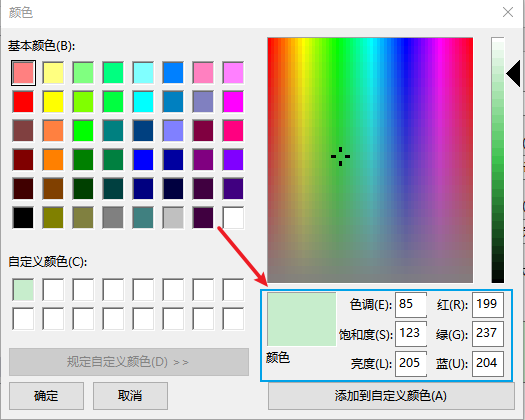如上图所示，这里没有能够直接输入十六进制颜色的选项
这个时候就需要把十六进制转换成十进制

利用Win10自带的计算器把十六进制转换成十进制：
首先在Win10的底部搜索框中输入“calc”或者中文“计算器”都可以找到Win10自带的计算器应用程序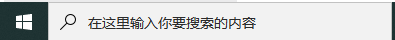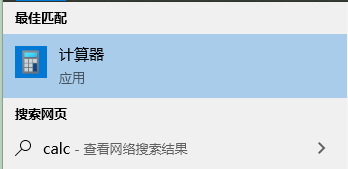打开计算器应用程序后，点击左上角的菜单按钮：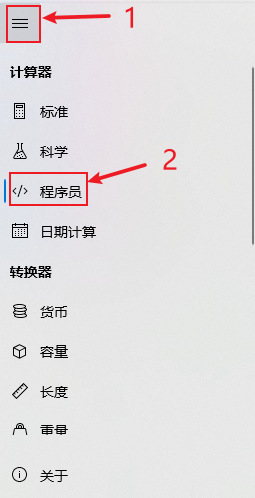选择“程序员”即可进入对应的专栏：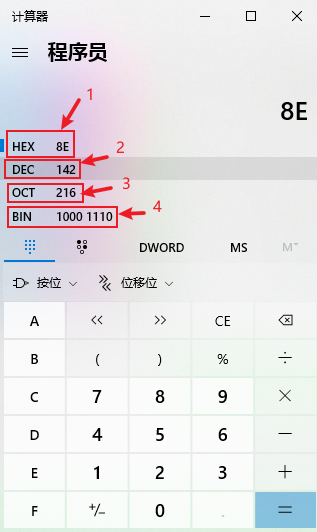如上图所示，分别对应：1 ——》十六进制，2 ——》十进制， 3 ——》八进制， 4 ——》二进制
当需要转换进制时，首先选择原来数据对应的进制，然后直接输入该数值即可。
比如需要转换十六进制"#C7EDCC"，则首先选择以上的“1” ——》“HEX"，然后在下方输入"C7"，即可得到下图：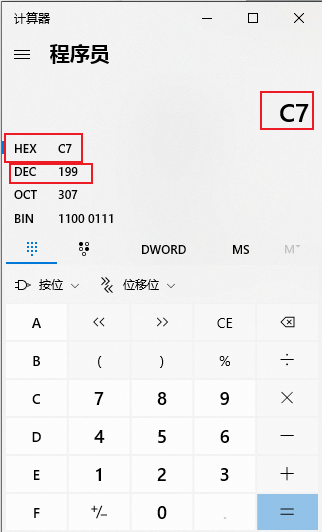从上图可知“C7”对应的十进制为“199”
用同样的方法可以得到“ED","CC"对应的十进制数值
注意：不要直接输入"C7EDCC"，因为这个颜色值对应的是“RGB”三个分量的数值，并不是统一的一个数值，所以需要将其分拆成“C7" —— "R"，"ED" ——“G”，“CC” —— "B”

八进制、二进制转换成十进制也是一样的方法，选择对应的选项即可切换

以上就是这些了，大家有问题可以留言交流哈，呵呵呵


展开全文css html
• 下载 Flurry 的外部 Jar ... 二进制计算器（在第 2 版中） - 基本数学运算 (+,-,/,x) 可能会添加更复杂的计算（例如 sin、cos、tan） 二元测验（完成） - 随机生成数（十进制到二进制）和（二进制到十进制）（完Java
• 二进制、八进制、十进制计算器 你好！ 这是你第一次使用 Markdown编辑器 所展示的欢迎页。如果你想学习如何使用Markdown编辑器, 可以仔细阅读这篇文章，了解一下Markdown的基本语法知识。 新的改变 我们对Markdown...
前言
该计算器程序支持二进制、八进制、十进制之间的互相转换，同时也支持二进制、八进制、十进制的计算。但是需要注意的是：程序可能存在着些许错误，请体谅笔者的水平。笔者提供了程序的完整文件，包括程序源代码。 本程序提供了“面向对象”和“面向过程”两种设计思路。
界面效果图Calculator类的代码
去博客设置页面，选择一款你喜欢的代码片高亮样式，下面展示同样高亮的 代码片.
using System;
using System.Collections.Generic;
using System.Linq;
using System.Text;

namespace MyCalculator
{
public class Calculator
{
//进制类型的枚举
public enum _bases { bin = 2, oct = 8, dec = 10 }
//标志当前运算进制，默认为十进制
public _bases _currentBase = _bases.dec;

//运算符的枚举
public enum _operators { add = 1, subtract = 2, multiply = 3, divide = 4}
//运算符默认为“+”

//进行各种进制下的各种运算
public string calculate(string num1, string num2)
{
string result;
//如果是二进制
if(_currentBase == _bases.bin)
{
else if (_operator == _operators.subtract)
result = BinSubtract(num1, num2);
else if (_operator == _operators.multiply)
result = BinMultiply(num1, num2);
else
result = BinDivide(num1, num2);
}
//如果是八进制
else if (_currentBase == _bases.oct)
{
else if (_operator == _operators.subtract)
result = OctSubtract(num1, num2);
else if (_operator == _operators.multiply)
result = OctMultiply(num1, num2);
else
result = OctDivide(num1, num2);
}
//如果是十进制
else
{
else if (_operator == _operators.subtract)
result = DecSubtract(num1, num2);
else if (_operator == _operators.multiply)
result = DecMultiply(num1, num2);
else
result = DecDivide(num1, num2);
}

return result;
}

//二进制转八进制
public string BinToOct(string bin)
{
string oct;
oct = Convert.ToString(Convert.ToInt32(bin, 2), 8);

return oct;
}

//二进制转十进制
public string BinToDec(string bin)
{
string dec;
dec = Convert.ToInt32(bin, 2).ToString();

return dec;
}

//八进制转二进制
public string OctToBin(string oct)
{
string bin;
bin = Convert.ToString(Convert.ToInt32(oct, 8), 2);

return bin;
}

//八进制转十进制
public string OctToDec(string oct)
{
string dec;
dec = Convert.ToInt32(oct, 8).ToString();

return dec;
}

//十进制转二进制
public string DecToBin(string dec)
{
string bin;
bin = Convert.ToString(Convert.ToInt32(dec), 2);

return bin;
}

//十进制转八进制
public string DecToOct(string dec)
{
string oct;
oct = Convert.ToString(Convert.ToInt32(dec), 8);

return oct;
}

//二进制加法
public string BinAdd(string num1, string num2)
{
string result;
string num1Dec = BinToDec(num1);
string num2Dec = BinToDec(num2);
result = DecToBin(Convert.ToString(Convert.ToInt32(num1Dec) + Convert.ToInt32(num2Dec)));

return result;
}

//二进制减法
public string BinSubtract(string num1, string num2)
{
string result;
string num1Dec = BinToDec(num1);
string num2Dec = BinToDec(num2);
result = DecToBin(Convert.ToString(Convert.ToInt32(num1Dec) - Convert.ToInt32(num2Dec)));

return result;
}

//二进制乘法
public string BinMultiply(string num1, string num2)
{
string result;
string num1Dec = BinToDec(num1);
string num2Dec = BinToDec(num2);
result = DecToBin(Convert.ToString(Convert.ToInt32(num1Dec) * Convert.ToInt32(num2Dec)));

return result;
}

//二进制除法
public string BinDivide(string num1, string num2)
{
string result;
string num1Dec = BinToDec(num1);
string num2Dec = BinToDec(num2);
result = DecToBin(Convert.ToString(Convert.ToInt32(num1Dec) / Convert.ToInt32(num2Dec)));

return result;
}

//八进制加法
public string OctAdd(string num1, string num2)
{
string result;
string num1Dec = OctToDec(num1);
string num2Dec = OctToDec(num2);
result = DecToOct(Convert.ToString(Convert.ToInt32(num1Dec) + Convert.ToInt32(num2Dec)));

return result;
}

//八进制减法
public string OctSubtract(string num1, string num2)
{
string result;
string num1Dec = OctToDec(num1);
string num2Dec = OctToDec(num2);
result = DecToOct(Convert.ToString(Convert.ToInt32(num1Dec) - Convert.ToInt32(num2Dec)));

return result;
}

//八进制乘法
public string OctMultiply(string num1, string num2)
{
string result;
string num1Dec = OctToDec(num1);
string num2Dec = OctToDec(num2);
result = DecToOct(Convert.ToString(Convert.ToInt32(num1Dec) * Convert.ToInt32(num2Dec)));

return result;
}

//八进制除法
public string OctDivide(string num1, string num2)
{
string result;
string num1Dec = OctToDec(num1);
string num2Dec = OctToDec(num2);
result = DecToOct(Convert.ToString(Convert.ToInt32(num1Dec) / Convert.ToInt32(num2Dec)));

return result;
}

//十进制加法
public string DecAdd(string num1, string num2)
{
string result;
decimal num1Dec = Convert.ToDecimal(num1);
decimal num2Dec = Convert.ToDecimal(num2);
result = Convert.ToString(num1Dec + num2Dec);

return result;
}

//十进制减法
public string DecSubtract(string num1, string num2)
{
string result;
decimal num1Dec = Convert.ToDecimal(num1);
decimal num2Dec = Convert.ToDecimal(num2);
result = Convert.ToString(num1Dec - num2Dec);

return result;
}

//十进制乘法
public string DecMultiply(string num1, string num2)
{
string result;
decimal num1Dec = Convert.ToDecimal(num1);
decimal num2Dec = Convert.ToDecimal(num2);
result = Convert.ToString(num1Dec * num2Dec);

return result;
}

//十进制除法
public string DecDivide(string num1, string num2)
{
string result;
decimal num1Dec = Convert.ToDecimal(num1);
decimal num2Dec = Convert.ToDecimal(num2);
result = Convert.ToString(num1Dec / num2Dec);

return result;
}
}
}

完整文件下载地址
计算器
展开全文c#
• QT编写的进制转换工具，包含代码，可以实现32位二进制十进制，16进制相互转换，可以对二进制单独位修改Qt
• 基于单片机(AT89C51)的进制转换进制计算器 闲来无事，用手头51单片机做个进制计算器 成品功能：能实现十进制带负数、带小数点的加减乘除，不同进制之间的转换，同一进制之间的加减乘。 材料 AT89C51开发板要带4x...
基于单片机(AT89C51)的进制转换及进制计算器
闲来无事，用手头51单片机做个进制计算器
成品功能：能实现十进制带负数、带小数点的加减乘除，不同进制之间的转换，同一进制之间的加减乘。

材料
AT89C51开发板要带4x4矩阵按键，再带8个独立按键才够用用LCD18650显示杜邦线用keil4进行开发
开始制作。
制作过程

思考过程

首先要考虑的就是按键数量了：
十进制的加减乘除带符号和小数需要：0~9十个按键 + 加减乘除四个按键 + 加小数点符号两个按键 = 共16个按键。不同进制之间转换需要：0~F十六个按键 + 2 8 10 16进制四个按键 = 共20个按键进制之间加减乘需要：0~F十六个按键 + 2 8 10 16进制四个按键+ 加减乘三个按键 = 共23个按键 这些还不算上回撤键和模式选择等按键。所以设计的时候必须考虑好按键。
我就得出来如下方案：
用独立按键做为模式选择：三个按键，选择模式之后不能返回，如果要返回可以用复位键。这样几个功能之间相互不联系，没有影响，按键就够用了。然后十进制的加减乘除带符号和小数：用4x4按键做出来键盘既789+ 456- 123* 退格0=/ 用两个独立按键做小数点和符号按键。不同进制之间转换：4x4按键做0~F既0123 4567 89AB CDEF，独立按键四个做进制选择既 2 8 10 16进制。进制之间加减乘 ：4x4按键做0~F既0123 4567 89AB CDEF，独立按键四个做进制选择既 2 8 10 16进制，独立按键四个做加减乘。

硬件部分

直接放图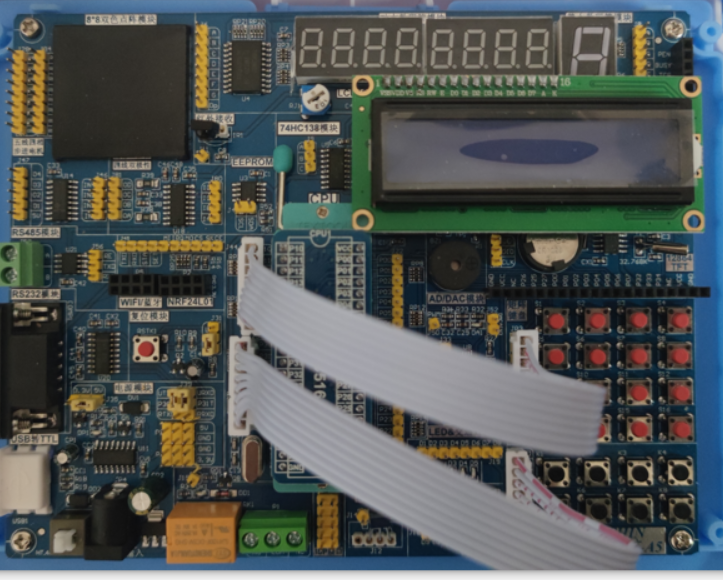如图所示连接P1接矩阵按键，P3接独立按键。
十进制的加减乘除带符号和小数
这里矩阵按键和独立按键怎么测值就不说了。

整数部分的加减乘除

将输入的数字连接起来，比如输入5、6，要连接成五十六，方法就是输入5存起来，再输入6，将存起来的数乘10再加上6存起来既五十加六，再输入7，再将存起来的数乘10再加上7存起来既五百六十加七。这样就将数字串起来了。按符号之前和按符号之后分别存到不同的变量中。当你按下等号之后，再去区别按下的符号是什么，让两次存的数做运算。
代码如下：
if(fuhao==1)//加
{
sprintf(Disp,"%-6.3f",a_l+b_l);	//将结果存在数组中方便显示
//spintf将右边的字符串到左边数组中
//%-6.3f为占位符既（1）表示靠左6位保留三位小数的浮点数
lcdwrd_string(Disp,6);	//显示
}
if(fuhao==2)//减
{
sprintf(Disp,"%-6.3f",a_l-b_l);
lcdwrd_string(Disp,6);
}
if(fuhao==3)//乘
{
sprintf(Disp,"%-9.4f",a_l*b_l);
lcdwrd_string(Disp,9);
}
if(fuhao==4)//除
{
sprintf(Disp,"%-9.4f",a_l/b_l);
lcdwrd_string(Disp,9);
}

这里直接显示运算结果，就避免了运算过程中产生的小数（两数相除）等数据类型问题。

负数问题

负数问题分情况来分析如果两个数相加，都是负号的话结果也有负号，都没有负号的话结果也没有负号；一正一负的话哪个数大结结果的符号就是哪个。两个数相减类似两个数相加。两个数相乘，看有奇数个还是偶数个负号，奇数个的话结果是负数，偶数个的话结果是正数。两数相除类似两数相乘。

小数问题

有小数点的话，我解决的方法是按完小数点之后记录小数点之后有几位，计算结果之前，让存起来的数乘0.1的多少次方。比如输入的3.1415，小数点之后有四位，存起来的数就是31415再四个0.1。
代码如下：
if(fhao_a==1){a_l = a*pow(0.1,a_wei)*(-1);}	 //将结果保存到double精度的数中
//有几位小数的话要乘0.1的几次方
//负数的话要成-1
else {a_l = a*pow(0.1,a_wei);}
if(fhao_b==1){b_l = b*pow(0.1,b_wei)*(-1);}
else {b_l = b*pow(0.1,b_wei);}

部分效果如图。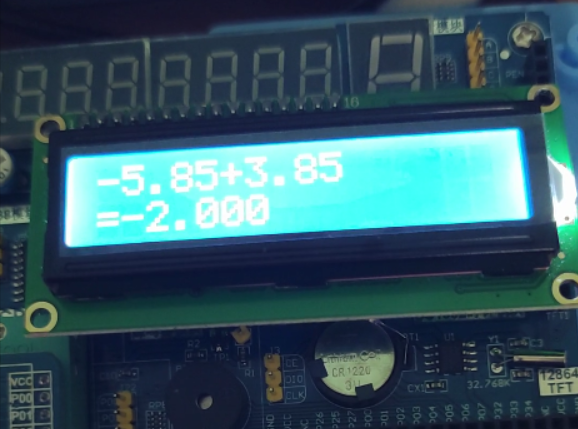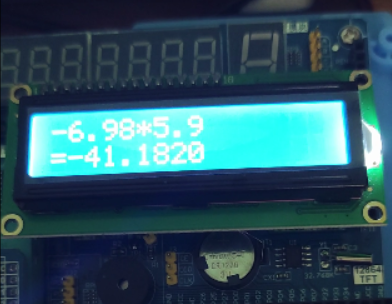这样十进制的加减乘除带符号和小数的问题就基本解决完了。
进制转换

思想

先将输入的数转换成十进制存起来。然后再按照要求输出不同进制的数。不同进制转10进制按权取数，比如：八进制的76，既6x8^0 +7x8^1 = 62。 例如二进制的代码：fhao_a = fhao_a*2 + k;10进制转不同进制除进制取余，比如：十进制62转八进制，62除8=7余数6，7除8=0余7，结果反过来写就是76。 例如二进制的代码： while(biao!=0) //一位一位显示 { lcdwrd(0x30+biao%2); biao=biao/2; }http://c.biancheng.net/view/142.html 可以参考这个网址。显示要将数倒过来显示，正好可以从右向左。 部分效果如图： 八进制转十六进制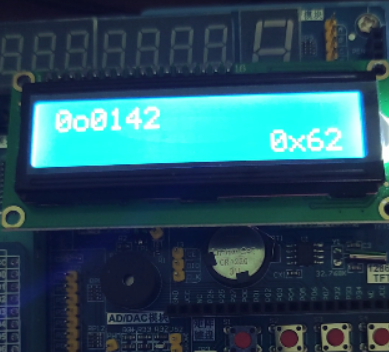八进制转二进制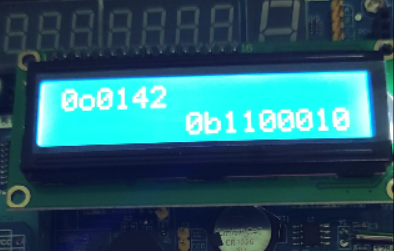十六进制转十进制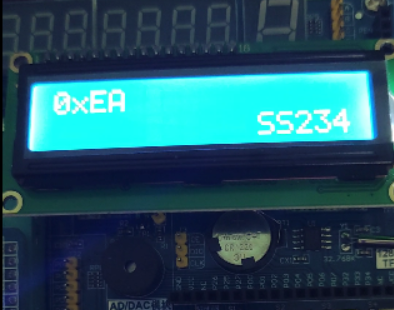这样进制转换问题就基本解决完了。
同一进制之间加减乘
为啥不做除呐，因为按键不够。一除就会出现小数，没想明白怎么表示。

思想还是以十进制保存，然后再做运算，再转化为相应进制的数输出。按键不够用所以没法做不同进制之间的运算。

效果如图所示： 十六进制减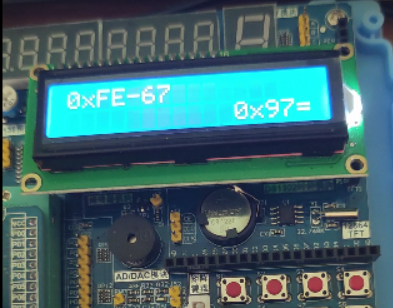二进制加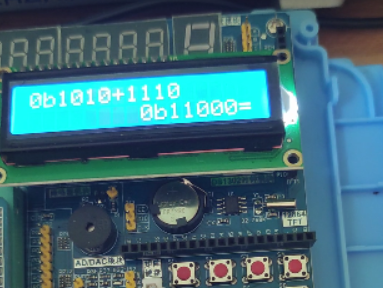这个项目到这里就算完了。 还有很多不足的地方，希望大佬可以指正。也希望和爱好者交流学习。
禁止转载！！！ QQ:2039723308 VX:Shiboven
展开全文• 语音计算器 含有二进制 八进制 十进制 十六进制转换 包含大小写输出
• 小数进制转换器，可以进行十进制小数转化为、八、十六进制
• MATLAB中负数8位二进制与10进制之间的转换MATLAB
• 个人原创软件，MFC界面，可以任意转换64bit数据到2进制、10进制、16进制，支持取位、移位、大小端转换、加、减、乘、除、取余、与、或、非计算，支持Unicode、ANSI、UTF-8编码相互...此版本支持二进制文本解析和复制
• [程序员二进制计算器 v1.36 帮助] 一 用法 在底部框中输入要计算的表达式，会即时显示计算结果，当无结果时说明表达式输入有误。 按回车键保存该次输入、清空输入框，并显示详细结果，包括可能有的错误信息。 ...
• 二进制由0和1组成，那么怎么快速的对一个十进制转换二进制数呢？ 最古老的方法大家还记得怎么转换吗？比如35这个十进制数？ 将这个数除以2，等于17余1，然后将17再除以2，等于8余1…直到不能再被2整除，然后将...算法
• 可以实现+，—，*，%，mod,sin,cos,tan,EXP,m+,m-,mr,mc,x^y 十进制二进制相互转换 换肤
• 按位运算符 按位与运算符 a & b 按位计算 a 和 b 的逻辑与 按位或运算符 a | b 按位计算a和b的逻辑或 按位异或运算符 a ^b 按位计算a和b的逻辑异或 ~运算符 ... int count = 0...